# 【数据结构与算法】一套链表 OJ 带你轻松玩转链表✨个人主页：bit me
✨当前专栏：数据结构
✨刷题专栏：基础算法

### 链 表 OJ

• 🏳️一. 移除链表元素
• 🏴二.反转链表
• 🏁三.链表的中间结点
• 🚩四.链表中倒数第k个结点
• 🏳️‍🌈五.合并两个有序链表
• 🏳️‍⚧️六.链表的回文结构
• 🏴‍☠️七.链表分割
• 🏴󠁧󠁢󠁷󠁬󠁳󠁿八.相交链表
• 🏳️‍🌈九.环形链表
• 🍹十.环形链表 II

# 🏳️一. 移除链表元素

①：列表中的节点数目在范围 [0, 104] 内
②：1 <= Node.val <= 50
③：0 <= val <= 50

①：时间复杂度O(n)
②：只遍历单链表一次

`所以我们在这里定两个指针cur和prev，其中cur代表的是要删除的节点，prev代表的是cur的前驱`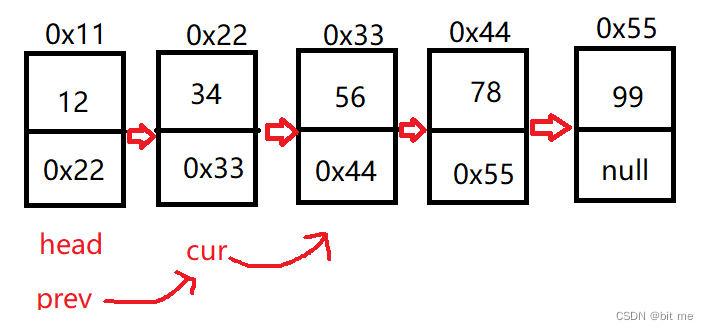1. 先考虑特殊情况：头节点不为空
``````if(head == null){
return null;
}
``````
1. 定义cur和prev指针位置
``````ListNode cur = head.next;
``````
1. 在删除节点之前，我们得知道cur走动的前提是不为空的
``````while(cur != null){
......
}
``````
1. 在cur 不为空的前提下，我们该如何删除元素呢？

``````if(cur.val == val){
prev.next = cur.next;
cur = cur.next;
}else{
prev = cur;
cur = cur.next;
}
``````
1. 最终代码就完成了，但是还有一个特殊情况，那就是cur是从第二个元素开始遍历，并没有考虑到头节点，所以我们在这里单独判断一下头节点
``````if(head.val == val){
}
``````

`附上总的代码：`

``````class Solution {
public ListNode removeElements(ListNode head, int val) {
return null;
}

while(cur != null){
if(cur.val == val){
prev.next = cur.next;
cur = cur.next;
}else{
prev = cur;
cur = cur.next;
}
}

//单独处理了头节点
}

}
}
``````

# 🏴二.反转链表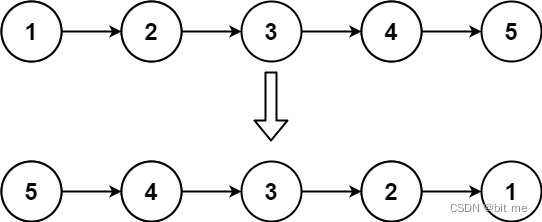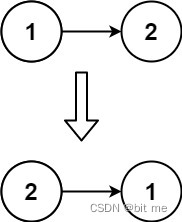①：链表中节点的数目范围是 [0, 5000]
②：-5000 <= Node.val <= 5000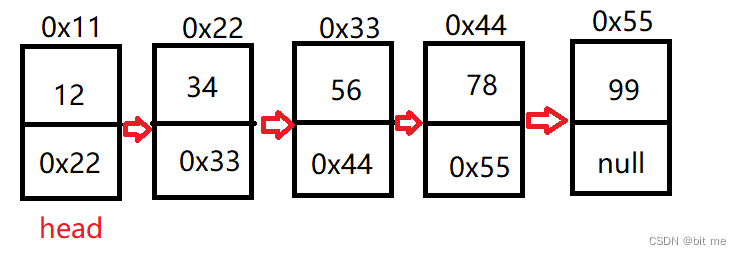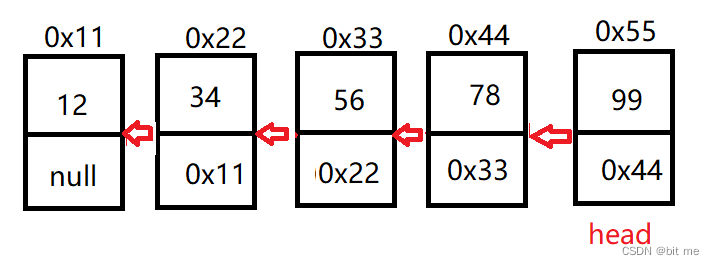`由上图可知，我们需要把头节点挪到最后面，让每个节点的指向发生反向改变，这时候我们就需要一个前驱信息来支撑我们的节点指向发生改变`

1. 先考虑特殊情况

①：当头节点为空的情况下直接返回空

``````if(head == null){
return null;
}
``````

②：只有一个节点

``````if(head.next == null){
}
``````
1. 根据图表直接把头节点的next设为null
``````head.next = null;
``````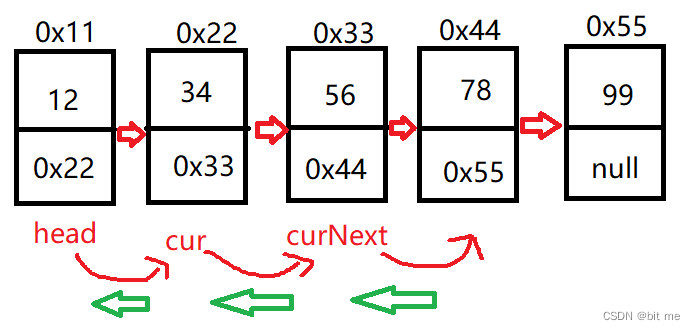``````ListNode cur = head.next;
``````

``````while(cur != null){
ListNode curNext = cur.next;
cur = curNext;
}
``````

`附上总的代码:`

``````class Solution {
return null;
}
//只有一个头节点
}

while(cur != null){
ListNode curNext = cur.next;
cur = curNext;
}
}
}
``````

# 🏁三.链表的中间结点

ans.val = 3, ans.next.val = 4, ans.next.next.val = 5, 以及 ans.next.next.next = NULL.

1. 对于求链表中间节点，最简单的想法就是链表遍历一次除2，但是按照我们上面要求来，是绝对不行的，有更好的解法是必要的
2. 我们只需要定义快慢指针就可以完成我们的需求，快指针是慢指针的两倍速度
3. 当节点数为奇数的时候，快慢指针一起走，当快指针fast.next == null的时候，慢指针slow为中间节点
4. 当节点数为偶数的时候，快慢指针一起走，当快指针fast == null的时候，慢指针slow为中间节点

原理就是当路程一定的时候，速度如果是二倍，当快的走到最后的时候，慢的一定在路程中间位置

``````public ListNode middleNode(){
while(fast != null && fast.next != null){
fast = fast.next.next;
slow = slow.next;
}
return slow;
}
``````

fast为空的话，fast.next就发生空指针异常了

# 🚩四.链表中倒数第k个结点

1. 此出照样使用快慢指针
2. 找出这里快慢指针的规律及判断条件
3. 例如我们要找的 k = 4 ，总的节点数为5个，这时候就可以让快指针先走，慢指针后走，慢指针所指向的位置就是k，所以当慢指针走到2，快指针走到了5，快指针就达到了临界条件停止了，返回了慢指针的位置，此时快指针比慢指针快3步，以此类推，快指针比慢指针快 k - 1 步的时候，当快指针遇到空，此时慢指针所指的位置就是第k个节点
1. 先考虑特殊情况（k的合法性和头节点）
``````if(k <= 0 ){
return null;
}
return null;
}
``````

1. 定义快慢指针，并且执行快指针比慢指针多的步数
``````ListNode fast = head;
while(k - 1 > 0){
fast = fast.next;
if(fast == null){
return null;
}
k--;
}
``````
1. 当快指针比慢指针快了 k - 1 步的时候，两个指针一起走，直至快指针达到临界条件
``````while (fast.next != null){
fast = fast.next;
slow = slow.next;
}
``````

`附上总的代码:`

``````public ListNode findKthTOTail(int k){
if(k <= 0 ){
return null;
}
return null;
}

while(k - 1 > 0){
fast = fast.next;
if(fast == null){
return null;
}
k--;
}
//fast已经走了k-1步了
while (fast.next != null){
fast = fast.next;
slow = slow.next;
}
return slow;
}
``````

# 🏳️‍🌈五.合并两个有序链表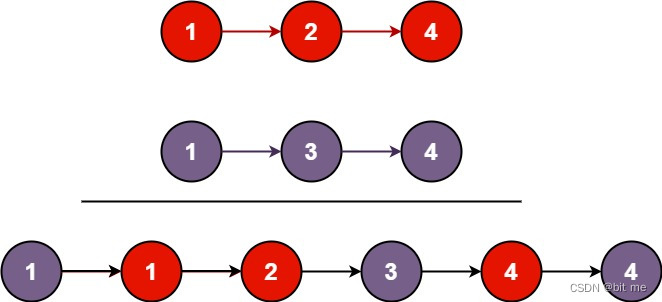1. 两个链表的节点数目范围是 [0, 50]
2. -100 <= Node.val <= 100
3. l1 和 l2 均按 非递减顺序 排列

1. 先考虑特殊情况，任何一链表为空
``````if (head1 == null) return head2;
``````
1. 定义一个新的链表来接收俩链表的合成，并且定义一个新的指针完成比较大小的走动来遍历打印新的链表
``````ListNode newHead = new ListNode(-1);
``````

``````while(head1 != null && head2 != null){
tmp = tmp.next;
}else {
tmp = tmp.next;
}
}
``````
1. 在这里我们还有个特殊情况，当任意一个链表走完之后，我们另外的一个链表就不用比较了，直接把剩下的节点拼接上去就可以
``````if(head1 != null){
}
}
``````

`附上总的代码:`

``````public ListNode mergeTwoLists(ListNode head1, ListNode head2) {

tmp = tmp.next;
}else {
tmp = tmp.next;
}
}
}
}
}
``````

# 🏳️‍⚧️六.链表的回文结构

1->2->2->1

1. 考虑特殊情况，链表为空或者只有一个头节点
``````if(this.head == null) return false;
if (this.head.next == null) return true;//只有一个节点
``````
1. 找中间节点
``````ListNode fast = head;
while(fast != null && fast.next != null){
fast = fast.next.next;
slow = slow.next;
}
``````
1. 反转后半部分链表
``````ListNode cur = slow.next;
while(cur != null){
ListNode curNext = cur.next;
cur.next = slow;
slow = cur;
cur = curNext;
}
``````
1. slow走到最后一个节点的时候，往中间遍历，此时头节点往中间遍历，看两边分别遍历是否相同，判断是否为回文串
``````while (head != slow){
return false;
}
//偶数的情况
return true;
}
slow = slow.next;
}
``````
2. 偶数的情况下，当遍历到相邻的时候，，判断是否是相引用即可

`附上总的代码:`

``````public boolean chkPalindrome() {
if (this.head.next == null) return true;//只有一个节点

//1.找中间节点
while(fast != null && fast.next != null){
fast = fast.next.next;
slow = slow.next;
}
//2.slow一定是中间节点,再翻转
ListNode cur = slow.next;
while(cur != null){
ListNode curNext = cur.next;
cur.next = slow;
slow = cur;
cur = curNext;
}
//3.slow走到了最后一个节点的地方
return false;
}
//偶数的情况
return true;
}
slow = slow.next;
}
return true;
}
``````

# 🏴‍☠️七.链表分割

x的值也可以是链表中没有的值

1. 考虑特殊情况
``````if(head == null) return null;
``````
1. 定义两条链表的首尾节点
``````ListNode bs = null;
ListNode be = null;
ListNode as = null;
ListNode ae = null;
``````
1. 遍历链表，然后进行分类，判断是分类到哪一条新链表中（尾插）
``````ListNode cur = head;
while(cur != null){
if(cur.val < x){
//判断是不是第一次插入
if(bs == null){
bs = cur;
be = cur;
}else {
be.next = cur;
be = be.next;
}
}else {
if(as == null){
as = cur;
ae = cur;
}else {
ae.next = cur;
ae = ae.next;
}
}
cur = cur.next;
}
``````
1. 判断元素大小然后进行分类，小于x的节点放在 bs – be 这条链表上，大于x的节点放在 as – ae 这条链表上
2. 每个链表第一次插入是特殊情况，要特殊处理
3. 除了第一个元素之外，我们再进行尾插的时候让 be，ae 走动，因为它俩是尾节点
1. 拼接两个新链表
``````if(bs == null){
//说明第一个区间没有数据
return as;
}
be.next = as;
if(as != null){
ae.next = null;
}
return bs;
``````

`附上总的代码:`

``````public ListNode partition(int x) {
ListNode bs = null;
ListNode be = null;
ListNode as = null;
ListNode ae = null;

while(cur != null){
if(cur.val < x){
//判断是不是第一次插入
if(bs == null){
bs = cur;
be = cur;
}else {
be.next = cur;
be = be.next;
}
}else {
if(as == null){
as = cur;
ae = cur;
}else {
ae.next = cur;
ae = ae.next;
}
}
cur = cur.next;
}
if(bs == null){
//说明第一个区间没有数据
return as;
}
be.next = as;
if(as != null){
ae.next = null;
}
return bs;
}
``````

# 🏴󠁧󠁢󠁷󠁬󠁳󠁿八.相交链表

1. intersectVal - 相交的起始节点的值。如果不存在相交节点，这一值为 0
2. listA - 第一个链表
3. listB - 第二个链表
4. skipA - 在 listA 中（从头节点开始）跳到交叉节点的节点数
5. skipB - 在 listB 中（从头节点开始）跳到交叉节点的节点数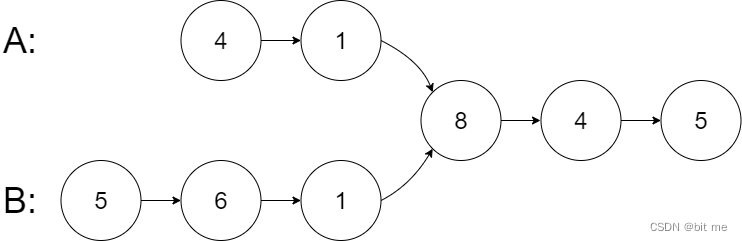— 请注意相交节点的值不为 1，因为在链表 A 和链表 B 之中值为 1 的节点 (A 中第二个节点和 B 中第三个节点) 是不同的节点。换句话说，它们在内存中指向两个不同的位置，而链表 A 和链表 B 中值为 8 的节点 (A 中第三个节点，B 中第四个节点) 在内存中指向相同的位置。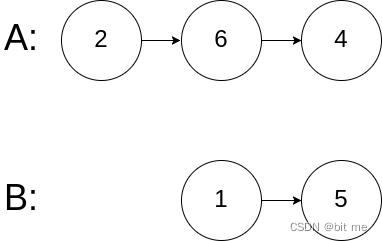1. listA 中节点数目为 m
2. listB 中节点数目为 n
3. 1 <= m, n <= 3 * 10^4
4. 1 <= Node.val <= 10^5
5. 0 <= skipA <= m
6. 0 <= skipB <= n
7. 如果 listA 和 listB 没有交点，intersectVal 为 0
8. 如果 listA 和 listB 有交点，intersectVal == listA[skipA] == listB[skipB]

1. 求两个链表的长度
2. 让长的链表先走差值步
3. 然后两个链表一起走，直到相遇
1. 特殊情况，有链表为空
``````if(headA == null || headB == null) return null;
``````
1. 遍历两个链表的长度
``````int lenA = 0;
int lenB = 0;

while(pl != null){
lenA++;
pl = pl.next;
}

while (ps != null){
lenB++;
ps = ps.next;
}
``````

1. 我们还需要用到pl，ps，所以这里还需要换回来
``````pl = headA;
``````
1. 差值步的计算和判断
``````//差值步 计算
int len = lenA - lenB;
if(len < 0){
len = lenB - lenA;//更新差值步
}
//走到这里，pl一定指向的是那个最长的链表，ps一定指向的是最短的链表，且len一定是一个正数

while (len != 0){
pl = pl.next;
len--;
}
//说明pl走了差值步了，接下来一起走直到他们两个相遇
``````

1. 差值步走完，俩链表一起走，直到相遇
``````while(pl != ps){
pl = pl.next;
ps = ps.next;
}
``````
1. 还要考虑特殊情况，没有交点的时候
``````if(headA == headB && headA == null){
return null;
}
``````

`附上总的代码:`

``````public ListNode getIntersectionNode(ListNode headA, ListNode headB) {

//1，求俩个链表的长度
int lenA = 0;
int lenB = 0;

while(pl != null){
lenA++;
pl = pl.next;
}

while (ps != null){
lenB++;
ps = ps.next;
}

//差值步 计算
int len = lenA - lenB;
if(len < 0){
len = lenB - lenA;//更新差值步
}
//走到这里，pl一定指向的是那个最长的链表，ps一定指向的是最短的链表，且len一定是一个正数

while (len != 0){
pl = pl.next;
len--;
}
//说明pl走了差值步了，接下来一起走直到他们两个相遇
while(pl != ps){
pl = pl.next;
ps = ps.next;
}

//说明没有交点
return null;
}
return pl;
}
``````

# 🏳️‍🌈九.环形链表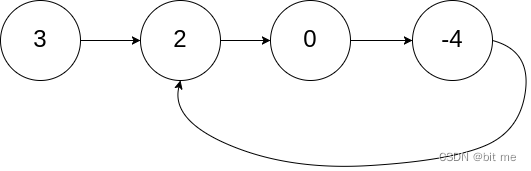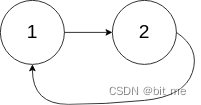1. 链表中节点的数目范围是 [0, 10^4]
2. -10 ^ 5 <= Node.val <= 10 ^ 5
3. pos 为 -1 或者链表中的一个 有效索引 。

1. 为什么快指针每次走两步，慢指针走一步可以？

1. 快指针一次走3步，走4步，…n步行吗？

`附上总的代码:`

``````public boolean hasCycle(ListNode head) {
while(fast != null && fast.next != null){
fast = fast.next.next;
slow = slow.next;
if(fast == slow){
return true;
}
}
return false;
}
``````

# 🍹十.环形链表 II1. 链表中节点的数目范围在范围 [0, 10^4] 内
2. -10 ^ 5 <= Node.val <= 10 ^ 5
3. pos 的值为 -1 或者链表中的一个有效索引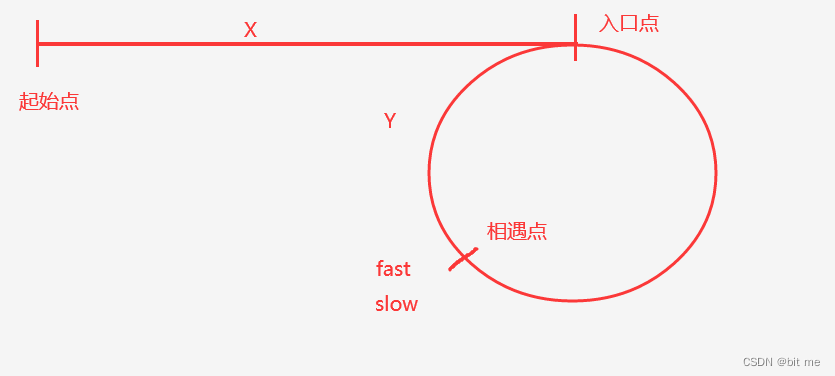1. 起始点到入口点的距离为X
2. 环的长度设置为C
3. 设相遇点到入口的距离为Y

fast只走了一圈的情况下：

slow走的路程：X + C - Y

fast走的路程：X + C + C - Y

fast走了n圈：

slow走的路程：X + C - Y

fast走的路程：X + NC + C - Y

1. 考虑特殊情况
``````if(head == null) return null;
``````
1. 定义快慢指针，并且由总结可知，相遇的时候需要另外挪动指针
``````ListNode fast = head;

while(fast != null && fast.next != null){
fast = fast.next.next;
slow = slow.next;
if(fast == slow){
break;
}
}
``````
1. break之后有两种情况，一是不满足循环，二是遇到break;说明是环且相遇
``````if(fast == null || fast.next == null){
return null;
}
while(slow != fast){
fast = fast.next;
slow = slow.next;
}
``````

`附上总的代码:`

``````public ListNode detectCycle(ListNode head) {

while(fast != null && fast.next != null){
fast = fast.next.next;
slow = slow.next;
if(fast == slow){
break;
}
}
//走到这里说明遇到俩种情况
//1.不满足循环，有一个为空
//2.遇到break;说明是环且相遇
if(fast == null || fast.next == null){
return null;
}